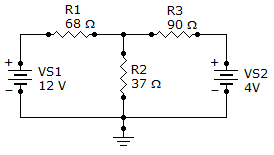# Electrical Engineering - Branch, Loop and Node Analyses - Discussion

Discussion Forum : Branch, Loop and Node Analyses - General Questions (Q.No. 3)
3.
Find branch current IR2.5.4 mA
–5.4 mA
113.0 mA
119.6 mA
Explanation:
No answer description is available. Let's discuss.
Discussion:
14 comments Page 2 of 2.

SURYA said:   8 years ago
(Va-12)/68)+((Va-0)/37)+((Va-4)/90) = 0.

(1665(Va-12)+3060Va+1258(Va-4))/113220 = 0.

1665Va-19980+3060Va+1258Va-5032 = 0.

5983Va = 25012.

So Va = 25012/5983 = 4. 1805 Volt but the current through the r2 resistor is 4.

180/37 = 113mA.

Nisar Ahmad Stanikzai said:   8 years ago
I=112.9mA.